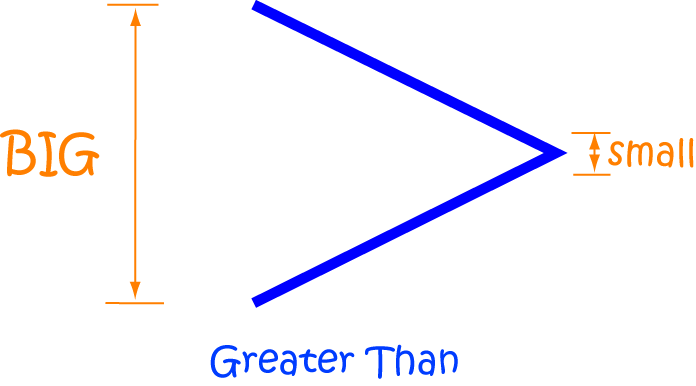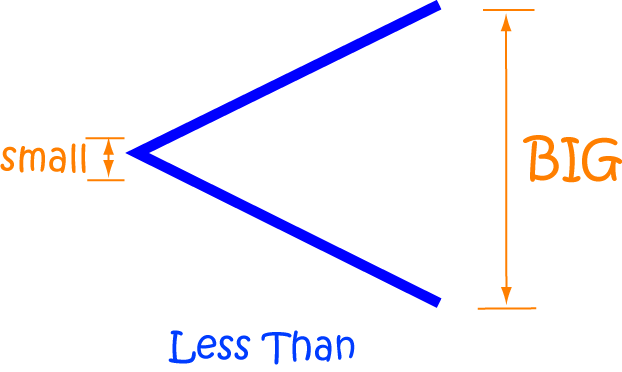# What are Inequalities?

We use inequalities when we want to compare the values of two numbers. In mathematics, we don't always know what things are equal to, but we may be able to get an idea of when something is greater than another number, or less than another number.

For example, if Jacinta notices that her older brother Aaron is allowed to watch more television than she is, she could complain that the amount of television she is allowed to watch is less than the amount of television that Aaron is allowed to watch, or that the amount of television that Aaron is allowed to watch is greater than the amount of television that she is allowed to watch.

If $j$ stands for the amount of television that Jacinta is allowed to watch, and $a$ stands for the amount of television that Aaron is allowed to watch, then we could write this as the inequality:

$j where "\( \(a > j$,
where "$>$" means "greater than."

We call these expressions inequalities because the things they are comparing don't have to be "equal".

## Greater Than and Less Than

The inequalities that are most commonly used are:

Symbol In Words Example
$>$ Greater Than $7 > 6$
$Less Than \(3 It looks like it could be difficult to remember which one is the "less than" symbol and which one is the "greater than" symbol, but there's an easy trick that helps us to remember: the "BIG" end of the symbol always opens out towards the bigger number, and the "small" end of the symbol always points towards the smaller number. For the greater than symbol, BIG \(>$ small .For the less than symbol, small $.### Example Jacinta goes for a ride on the roller coaster at Luna Park. The sign tells her that she must be over 120 cm to ride. How tall is Jacinta? We don't know exactly how tall Jacinta is, but we do know something about her height. It must be "over 120 cm", so we can write down an inequality for Jacinta's height: \(\text{Height} > 120 \text{ cm}$,
The big end opens out towards "Height" because Jacinta's height must be greater than 120 cm.

## Greater Than or Equal to and Less Than or Equal to

Sometimes inequalities allow values to be equal. For example, your mum might insist than you eat 3 or more brussels sprouts for dinner. We don't know how many brussels sprouts you will eat, but we do know that the number will equal at least 3 (OK, maybe we do know how many you'll eat ...).

To write these inequalities, we need to use symbols that include both an equals sign and a less than or greater than symbol. These symbols are described below.

Symbol In Words Example
$\ge$ Greater Than or Equal to $7 \ge 7$ and $7 \ge 6$
$\le$ Less Than or Equal to $3 \le 3$ and $3 \le 12$

### Example:

Aaron opens a social media account. You need to be 13 or older to open an account.

In this case, we know that Aaron's age must be more than or equal to 13 years. We can express this as an inequality as follows:

$\text{Age} \ge 13$.

### Another example:

Megan goes to the zoo with her parents. They don't have to pay for her ticket as children aged up to 5 years old get in for free. How old is Megan?

Again, we don't know exactly how old Megan is, but we do know that the oldest she can be is 5. We can express this as:

$\text{Age} \le 5$.
Here, Megan's age is the small number, so the pointy bit of the symbol is directed towards "Age."

You can practise comparing the ages, heights and weights of your friends using 

If you'd like to read more about inequalities, check out the article on comparing numbers.

### Description

This mini book covers the core of Math for Foundation, Grade 1 and Grade 2 mathematics including

1. Numbers
3. Subtraction
4. Division
5. Algebra
6. Geometry
7. Data
8. Estimation
9. Probability/Chance
10. Measurement
11. Time
12. Money
13. and much more

This material is provided free of cost for Parent looking for some tricks for their Prekinder, Kinder, Prep, Year 1 and Year 2 children

### Learning Objectives

These lessons are for kids aged 4-8 with the core objective to expose their brains to concepts of addition, subtraction, division, algebra and much more.

Author: Subject Coach
You must be logged in as Student to ask a Question.